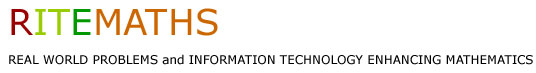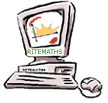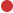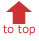Project Home Publications & Seminars Research Materials Project Team Only

Linear Function ResourcesBarbie Bungee Task Modeling task, typically used at end of topicGraphic Algebra Linear Functions Guide to learning based in real world contexts - supported by function grapherLinear Functions and Measurement Links between linear function graphs triangular areasRules from patterns Example problem: flowerbedFocus on gradient Explore gradient: spreadsheet & dynamic geometryPipe Problem Problem uses both linear & quadratic functions

In this task students collect data linking the number of rubber bands attached to a 'Barbie Doll' with the distance that the Doll will fall, and then fit a linear model to this data to predict the number of bands needed so the Doll can be dropped from a given height without hitting the floor.

BarbieBungeeActivitySheet (pdf) - student sheet that introduces and guides students through the Barbie Bungee Task; students use spreadsheet and/or graphics calculator

FitLineByEyeGSPSketch (gsp) - a Geometer's Sketchpad sketch on which students can plot collected data points, "fit a line by eye' to the plotted points and read the equation of this line; could be used as part of Barbie Bungee Task

Graphic Algebra Linear Functions

The book Graphic Algebra was written for students with access to computer / calculator graphing. The approach to linear functions from Chapter 1 of this book was successfully used with students at year 8/9 who had access to a graphing calculator or CAS calculator.

Tests - pre and post tests that were used in 2005 and 2006

 2005 PreTestLinearFunctions (pdf) 2006 PreTestLinearFunctions (pdf)

CAS Supplement - used to incorporate student use of CAS

GraphAlgCAS (pdf) - this sheet introduces some simple CAS use into the Graphic Algebra text for Chapter 1

CASLinearEqSolveSheet (pdf) - student activity sheet focussing on solving linear equations by "doing the both to both sides" with the aid of CAS

Linear Function Files - Rules from Patterns

The file below were created and used by Alan Bennetto at Westbourne Grammar School as part of a year 9 linear function unit

FlowerBedTaskSheet (pdf) - student task sheet using flower beds as context for students to find linear function rules from patterns in tables of data

Linear functions and Measurement

In this six-lesson unit of work for Year 10 students, the links between linear function graphs and the triangular areas they determine with the coordinate axes are explored. Multiple representations of the emerging quadratic relationships are analysed in specific and general cases.  Each lesson features a detailed lesson plan and a student worksheet, while background notes and lesson commentaries are provided for teachers. The unit utilises either TI-89 (OS v3.10) or TI-Nspire CAS (v1.6) technology as seen in the files below.

Two Spreadsheets and a Geometer's Sketchpad sketch, that demonstrate how the graph of the linear function y = mx+c depends on its slope (m) and y-intercept (c)
These files below were created and used by Alan Bennetto at Westbourne Grammar School as part of a year 9 linear function unit. They are suitable for class demonstrations or for students to use for guided exploration of the concept of gradient.

SlopeInterceptSpreadsheet (xls) - spreadsheet that allows the gradient and y-intercept for a line to be specified and produces a table of values and the graph of the line

SlopeInterceptGSPSketch (zipped gsp) - a Geometer's Sketchpad sketch that allows the gradient and y-intercept for a line to be specified, or automatically varied, and produces the graph of the line

Pipe Problem

The functional relationship between the two variables in this problem is a linear one, but a quadratic equation needs to be found and solved to find the rule for this linear relationship

PipeProbSheet (pdf) - student sheet with statement of problem and a static diagram

PipeProbGSPSketch (zipped gsp) - a Geometer's Sketchpad sketch with a page giving the problem and a diagram that can be changed, a second page for collecting and plotting data, third page for exploring the functional relationship between the variables in the problem, and finally a page showing the function rule; link buttons are used to move through the pages in sequence

PipeProbCAS (pdf) - a solution to the problem by use of CAS (TI-Interactive was used)